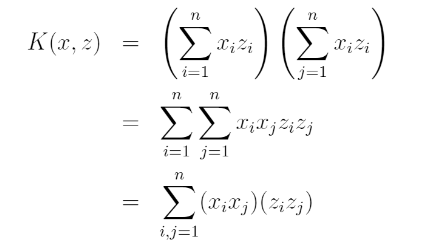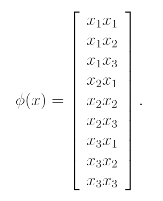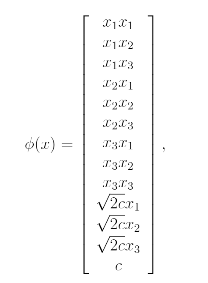# [Stanford CS229] Lecture7 - Kernels

jhbale11·2021년 7월 1일
1

## Stanford CS229 Lecture Note

목록 보기
7/8## 1. Kernels

In Linear regression, we had a problem in which the input x was the living area of a house and we considered performing regression using the features $x, x^2, x^3$ to obtain a cubic function. To distinguish betwwen these two sets of variables we'll call the original input value that input attributes of a problem.

When that is mapped to some new set of quantities that are then passed to the learning algorithm, we'll call those new quantities the input features. We will also let $\phi$ denote the feature mapping, which maps from the attributes to the features.

$\phi(x) = [x, x^2, x^3]$

Rather than applying SVMs using the original input attributes $x$, we may instead want to learn using some features $\phi(x)$. To do so, we simply need to go over our previous algorithm, and replace $x$ everywhere in it with $\phi(x)$.

Since Algorithm can be written entirely in terms of the inner products <x,z>, this means that we would replace all those inner products with $<\phi(x), \phi(z)>$. Specificially given a feature mapping $\phi$, we define the corresponding Kernel to be

$K(x,z) = \phi(x)^T \phi(z)$

Then everywhere we previously had <x,z> in our algorithm, we could simply replace it with K<x,z> and our algorithm would now be learning using the feature $\phi$.

We can also write it as this form:Thus, we see that $K(x,z) = \phi(x)^T \phi(z)$, where the feature mapping $\phi$ is given by위의 예시는 $x$의 n이 3인 경우를 표현한 것이며, 만약 $K(x,z) = (x^T z+c)^2$의 형태라면 feature mapping은 다음과 같을 것이다.이를 일반적으로 표현하자면 $K(x,z) = (x^T z+c)^d$의 경우에는 feature mapping은 (n+d)P(d)의 feature space를 가지게 될 것이지만 이 dimensional space가 $O(n^d)$이더라도 $K(x,z)$를 계산하는데는 여전히 $O(n)$ 만큼의 시간이 걸릴 것이다.

만약 x와 z가 비슷하다면 $K(x,z) = \phi(x)^T \phi(z)$는 클 것이며,
만약 x와 z가 비슷하지 않다면 $K(x,z) = \phi(x)^T \phi(z)$는 작을 것이다.
이는 feature mapping을 계산하는 과정에서 inner product가 이루어졌기 때문이다.

If you have any learning algorithm that you can write in terms of only inner products <x,z> between input attribute vectors, then by replacing this with K<x,z> where K is a kernel, you can "magically" allow your algorithm to work efficiently in the high dimensional feature space corresponding to K.